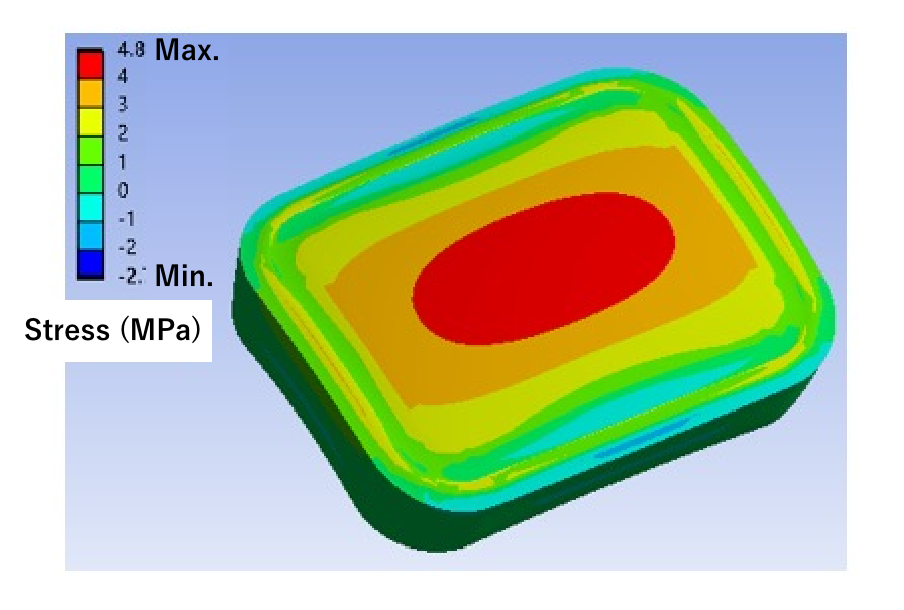Infrasctructure

# Design & Simulation

## Reactor design and simulations

Numerical simulations using high performance computers play an important role in scaling up reactors.
Our simulation technology comprises three main components: electromagnetic field, thermal fluid dynamics, and structural analyses.
We employ coupled analysis, a complex technique that combines multiple methodologies depending on the objective. The various software packages we use in simulations are shown below.

### Simulation software used at MWCC

Target of analysis Software use
Electromagnetic field Ansys HFSS
CST Studio Suite
Thermal fluid dynamics Ansys Fluent
Ansys Rocky
Structural analysis Ansys Mechanical
Multiphysics simulations COMSOL Multiphysics

## Electromagnetic field analysis

Electromagnetic field analysis lets us solve Maxwell’s equations and visualize both how microwaves propagate through the reactor and the calorific value distribution within the molecule being heated with microwaves. The complex dielectric constant we measure is then assigned to the heated object. We use different software to suit the scale of each study, i.e., lab, bench, or pilot.

• ### Ansys HFSS

This is used to analyze reactors from lab to bench and pilot scale to commercial scale reactors up to a certain size. It shows how the electromagnetic fields behave for each wavelength (frequency-domain analysis).

Fig. 1:Electromagnetic field strength distribution within a microwave reactor

• ### CST Studio Suite

This software is used in analyzing reactors at the pilot scale thanks to the relatively small amount of memory it requires. In addition to frequency-domain analysis, it can calculate electromagnetic field behavior over time (time-domain analysis). It also supports coupled analysis of electromagnetic fields and thermal fluid dynamics.Fig. 2:Microwave heat distribution in a pilot scale stirring tank

## Thermal fluid dynamics analysis

• ### Ansys Fluent

Thermal fluid dynamics analysis solves fluid continuity equations, equations of motion, energy equations, and similar equations, including reaction formulas if required. This allows us to understand temperature changes in the heated molecule, as well as the progress of chemical reactions and the movement of liquids inside the reactor.Fig. 3:The trajectory of liquid movements inside a reactor

• ### Ansys Rocky

This uses the Discrete Element Method (DEM) to solve particle motion equations when examining the behavior of granular materials, typically catalytic particle systems.Fig. 4:Velocity distribution of gases and particles in a fluid bed

## Structural analysis

• ### Ansys Mechanical

Structural analysis calculates the values for the strain and stress generated within the casing from rising pressure inside a reactor. The results of these analyses are used to determine the strength of the casing.Fig. 5:Distribution of stress generated in a planar object

## Multiphysics simulations

• ### COMSOL Multiphysics

COMSOL Multiphysics® is a general-purpose finite element method (FEM) physics simulation software designed to perform multiphysics simulations. It can calculate the distribution of electromagnetic fields using parameters obtained from complex permittivity measurements and then use those results to calculate down to heat distribution analysis, all within one program. As the name implies, this tool is helpful in considering and connecting the behavior of electromagnetic waves and exothermic behavior in reaction systems with chemical reactions.Fig. 6:The electromagnetic field distribution of a ceramic sample placed in a development microwave reactor